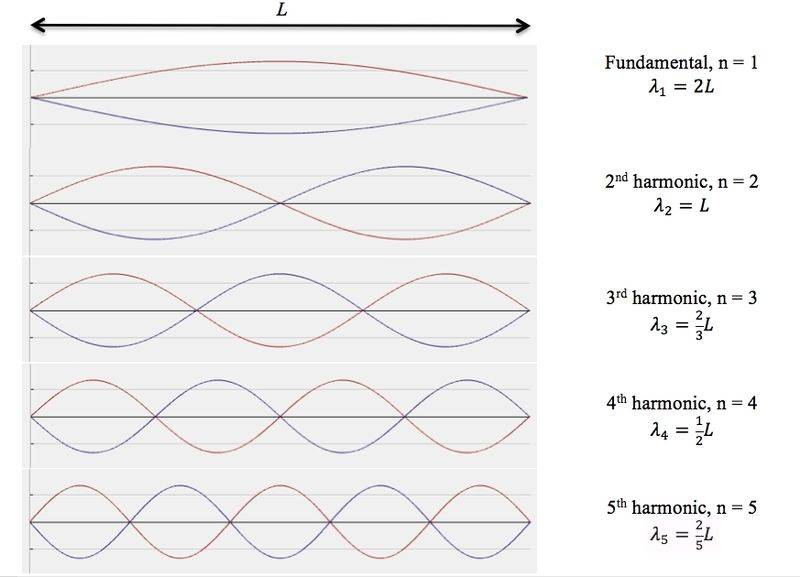# Physics - Resonance

• ElegantSir

#### ElegantSir

A string that is 6m long is vibrating with 3 loops in it. The frequency of the source is 16.5Hz.
What is the fundamental frequency of the string?

For the life of me I can't figure out how to solve this question. I found out that the wavelength is 4.8m but that's about it. If I use the equation fn = nf1 this is all I seem to be getting when I plug in the numbers. fn = 1 * 16.5Hz which is still equal to 16.5Hz...Id like to know what I am missing here.

A string that is 6m long is vibrating with 3 loops in it. The frequency of the source is 16.5Hz.
What is the fundamental frequency of the string?

For the life of me I can't figure out how to solve this question. I found out that the wavelength is 4.8m but that's about it. If I use the equation fn = nf1 this is all I seem to be getting when I plug in the numbers. fn = 1 * 16.5Hz which is still equal to 16.5Hz...Id like to know what I am missing here.

Please stop deleting the Homework Help Template. It is important for you to start your schoolwork threads using that template. For example, what are the "Relevant Equations" for this type of question?

Also, when there are "3 loops", what does that look like? Can you post a figure or a sketch? And when the string is vibrating at its fundamental frequency, what does that look like?

•DEvens
I apologize for that.

The equations that I have been given to use for this unit are:
fn = nf1 - n being the nunber of cycles and f1 being the frequency
L1 = λ / 4 - L1 being the resonant wavelength
v = fλ
v = d / t

There are no figures or sketches for the question, I am strictly just going off of the numbers that it has given me.
All I know about the fundamental frequency in regards to the question is that it is the lowest possible frequency that I am trying to find, using the equation fn = nf1 to find it.

I apologize for that.

The equations that I have been given to use for this unit are:
fn = nf1 - n being the nunber of cycles and f1 being the frequency
L1 = λ / 4 - L1 being the resonant wavelength
v = fλ
v = d / t

There are no figures or sketches for the question, I am strictly just going off of the numbers that it has given me.
All I know about the fundamental frequency in regards to the question is that it is the lowest possible frequency that I am trying to find, using the equation fn = nf1 to find it.

Well, it's pretty easy to use Google Images to find figures that can help you figure this out. I did a Google Images search on string harmonics, and got lots of figures like this one:Count the little loop thingies...